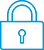cancel
Showing results for
Did you mean:It has been a while since anyone has replied. Simply ask a new question if you would like to start the discussion again.New member
3 1 0 0
Message 1 of 4
551
Flag Post

# Hp prime desolve polynomialHP Recommended
HP PRİME G2

I have a problem, problem is desolve

i write desolve(x*y''+(x-1)*y'-y=x^2,y) on the g2 but its cannot this , i get warning polynomial solution found x-1 and

i get anwser

y=(x-1)*((-G_0*e^(-x)+x^2-x+1)/(x-1)+G_1) but this is not true.

True is the y=(x^2+(c1-2)*x)+2-c1+c2*e^(-x)

Tags (5)
3 REPLIES 3Level 3
28 26 1 5
Message 2 of 4
Flag PostHP Recommended

If you use expand, and then collect, to rewrite the Prime's solution you'll see it is basically the same expression.

Jurjen Bos
Calculator nerd since 1980. Cryptographer by profession.New member
3 1 0 0
Message 3 of 4
Flag PostHP Recommended

I didn't find same solution i tried to your solve but didnt workLevel 3
28 26 1 5
Message 4 of 4
Flag PostHP Recommended

Let me be a bit more clear. I press CAS first, entering in textbook mode (which I show here as algebraic, directly copied and pasted from the virtual calculator).

If you give desolve(x*y''+(x-1)*y'-y=x^2,y), you get as result (x-1)*((-G_0*e^(-x)+x^2-x+1)/(x-1)+G_1)

If you enter expand(Ans), you get G_1*x+x^3/(x-1)-2*x^2/(x-1)+2*x/(x-1)+G_0*e^(-x)/(x-1)-G_0*x*e^(-x)/(x-1)-G_1-1/(x-1) which doesn't look like progress yet.

Now enter collect(Ans) to get -G_0*e^(-x)+G_1*x+x^2-G_1-x+1 and this effectively the solution you gave.

Jurjen Bos
Calculator nerd since 1980. Cryptographer by profession.
† The opinions expressed above are the personal opinions of the authors, not of HP. By using this site, you accept the Terms of Use and Rules of Participation